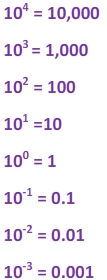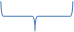# Scientific Notation of Small Numbers

Scientific Notation is a handy way to rewrite large numbers. For example, a number like 45,600,000,000 can be rewritten as 4.5 x 1010. But what happens when we have really tiny numbers?

The mass of a proton is
0.00000000000000000000000000167262178 kg.

This very small number is just as hard to work with as the very large numbers. So let's take a look at how scientific notation can be used to rewrite this number.

Take a look at the powers of ten:We can see that decimals can be rewritten using negative exponents. For example, if I wanted to write 0.0004, I can think of this as 4 x 0.0001 or we could use an exponent and say that this number is equal to 4 x 10-4. This is an example of scientific notation.

Remember, a number written in scientific notation has two parts:

# less than ten x power of ten

This first number needs to be greater than or equal to one as well. Nonetheless, lets put the rules to work with some examples.

Examples

1.)0.000003426

Step 1: Move the decimal so that there is only one digit in front of the decimal.

0.000003.426

Step 2: Count the number of moves from the original decimal to the new position.

0.000003.426There are 6 moves

Step 3: Write the new number as a product with a power of ten.

3.426 x 10-6 The number of moves becomes the exponent.

2.)0.00000000291

Step 1: Move the decimal between the 2 and 9.

0.000000002.91

Step 2:Count the number of moves from one decimal to the other.

0.000000002.91There are 9 moves.Step 3: Write the new number.

2.91 x 10-9

We can also change a number written in scientific notation back to standard form.

Take a look at how we can use the steps in the opposite order.

Examples:

1.) 5.8 x 10-3

Step 1: Take note of the exponent. The exponent tells us how many times we will move over.

5.8 x 10-3

Step 2: Move the decimal to the left 3 times because the exponent is a negative 3. Place zeros in the empty spots as you move.

.0058

0.0058

2.) 7 x 10-5

Step 1: The exponent is a negative 5.

Step 2: The decimal is located after the 7. Now it needs to move 5 places to the left.

.00007

Step 3: So the final answer is 0.00007.

Take note that exponent is the number of moves, not the number of zeros!

Let's Review:

When faced with a really small number, we can rewrite it so that there are less digits to work with by using powers of ten. The final answer should be a product with a number less than ten (but greater than or equal to one) times a power of ten. When your number in standard form is less than one, but greater than zero, you will have a negative exponent in your scientific notation.

5.6 x 10-4 = 0.00056